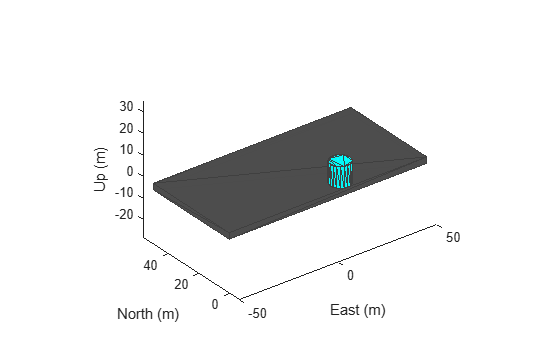Add new static mesh to UAV scenario

## Syntax

``addMesh(scene,type,geometry,color)``
``addMesh(___,Name,Value)``

## Description

````addMesh(scene,type,geometry,color)` adds a static mesh to the UAV scenario `scene` by specifying the mesh type, geometry, and color.```

example

````addMesh(___,Name,Value)` specifies additional options using name-value arguments. Enclose each `Name` in quotes.```

## Examples

collapse all

Create a UAV Scenario.

`scene = uavScenario("UpdateRate",100,"StopTime",1); `

Add the ground and a building as meshes.

```addMesh(scene,"Polygon", {[-50 0; 50 0; 50 50; -50 50], [-3 0]}, [0.3 0.3 0.3]); addMesh(scene,"Cylinder", {[10 5 5], [0 10]}, [0 1 1]); ```

Visualize the scenario.

`show3D(scene);`## Input Arguments

collapse all

UAV scenario, specified as a `uavScenario` object.

Mesh type, specified as `"cylinder"`, `"surface"`, `"terrain"`, `"polygon"`, or `"custom"`. Specify the geometric parameters of the mesh using the `geometry` input.

Data Types: `string`

Mesh geometry, specified as a cell array of geometry parameters. Depending on the `type` input, the geometry parameters have different forms:

`type` Input ArgumentGeometry ParametersDescription
`"cylinder"``{[centerx, centery, radius],[zmin, zmax]}``centerx` and `centery` are the x- and y-coordinates of the center of the cylinder, respectively. `radius` is the radius of the cylinder in meters. `zmin` and `zmax` are the minimum and maximum z-axis coordinates of the cylinder in meters, respectively.
`"surface"``{meshGridX,meshGridY,z}``meshGridX`, `meshGridY` and `z` are all 2-D matrices of the same size that define the xyz-points of the surface mesh.
`"terrain"``{terrainName,Xlimits,YLimits}`You must first call the `addCustomTerrain` function to load the terrain data and specify a terrain name. Specify the minimum and maximum xy-limits as two separate two-element vectors in local coordinates, or latitude-longitude coordinates if the `'UseLatLon'` name-value pair is `true`. The xy-coordinates must be specified in the ENU reference frame.
`"polygon"``{cornerPoints,[zmin, zmax]}``zmin` and `zmax` are the minimum and maximum z-axis coordinates of the polygon in meters, respectively. `conerPoints` contains the corner points of the polygon, specified as a N-by-2 matrix, where N is the number of corner points. The first column contains the x-coordinates and the second column contains the y-coordinates in meters.
`"custom"``{vertices,faces}``vertices` is an n-by-3 matrix of mesh points in local coordinates. `faces` is an n-by-3 integer matrix of indexes indicating the triangular faces of the mesh.

Mesh color, specified as a RGB triplet.

Example: `[1 0 0]`

### Name-Value Arguments

Specify optional comma-separated pairs of `Name,Value` arguments. `Name` is the argument name and `Value` is the corresponding value. `Name` must appear inside quotes. You can specify several name and value pair arguments in any order as `Name1,Value1,...,NameN,ValueN`.

Example: ```addMesh(scene,"Cylinder",{[46 42 5],[0 20]},[0 1 0],"UseLatLon",true)```

Enable latitude and longitude coordinates, specified as `true` or `false`.

• When specified as `true`, the x and y coordinates in the `geometry` input are interpreted as longitude and latitude, respectively.

• When specified as `false`, the x and y coordinates in the `geometry` input are interpreted as Cartesian coordinates.

Reference frame of the geometry input, specified as an inertial frame name defined in the `InertialFrames` property of the `uavScenario` object `scene`. You can add new inertial frames to the scenario using the `addInertialFrame` object function.

The scenario only accepts frames that have z-axis rotation with respect to the `"ENU"` frame.